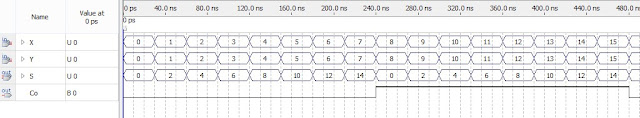# Verilog Code for Ripple Carry Adder

## The 4-bit ripple-carry adder is built using 4 1-bit full adders as shown in the following figure.#### Or use the structural Verilog code for the full adder based on its logic diagram as follows:#### Verilog code for 1-bit full adder using structural modeling:

```// fpga4student.com: FPGA projects, Verilog projects, VHDL projects
// Verilog project: Verilog code for 4-bit ripple-carry adder
// Verilog code for 1-bit full adder
module fulladder(X, Y, Ci, S, Co);
input X, Y, Ci;
output S, Co;
wire w1,w2,w3;
//Structural code for one bit full adder
xor G1(w1, X, Y);
xor G2(S, w1, Ci);
and G3(w2, w1, Ci);
and G4(w3, X, Y);
or G5(Co, w2, w3);
endmodule
```
Then, instantiate the full adders in a Verilog module to create a 4-bit ripple-carry adder using structural modeling.

### Following is the Verilog code for the 4-bit ripple-carry adder:

```// fpga4student.com: FPGA projects, Verilog projects, VHDL projects
// Verilog project: Verilog code for 4-bit ripple-carry adder
input [3:0] X, Y;// Two 4-bit inputs
output [3:0] S;
output Co;
wire w1, w2, w3;
// instantiating 4 1-bit full adders in Verilog
fulladder u1(X, Y, 1'b0, S, w1);
fulladder u2(X, Y, w1, S, w2);
fulladder u3(X, Y, w2, S, w3);
fulladder u4(X, Y, w3, S, Co);
endmodule
```
Now, it's time to run a simulation to see how it works. In this Verilog project, let's use the Quartus II Waveform Editor to create test vectors and run functional simulations without a Verilog testbench. If you want to learn how to run the simulation without a Verilog testbench, you can check the tutorial: here.

#### Below is the simulation waveform for the Ripple-Carry Adder in Verilog:The simulation waveform demonstrates the accurate functional operations of the 4-bit ripple-carry adder that is implemented in Verilog above.